# “EfficientNet: Rethinking Model Scaling for Convolutional Neural Networks” Summarized

Categories:

Updated:

https://arxiv.org/abs/1905.11946 (2019-06-10)

## 1. Introduction

EfficientNet group is composed of ConvNets EfficientNetB0~EfficientNetB7. (bigger number means more parameters) EfficientNetB7 achieved state of the art in ImageNet classification with considerably less parameters than previous SOTA, GPipe. Smaller EffficientNets also achieved higher accuracies than previous architectures that have similar complexities.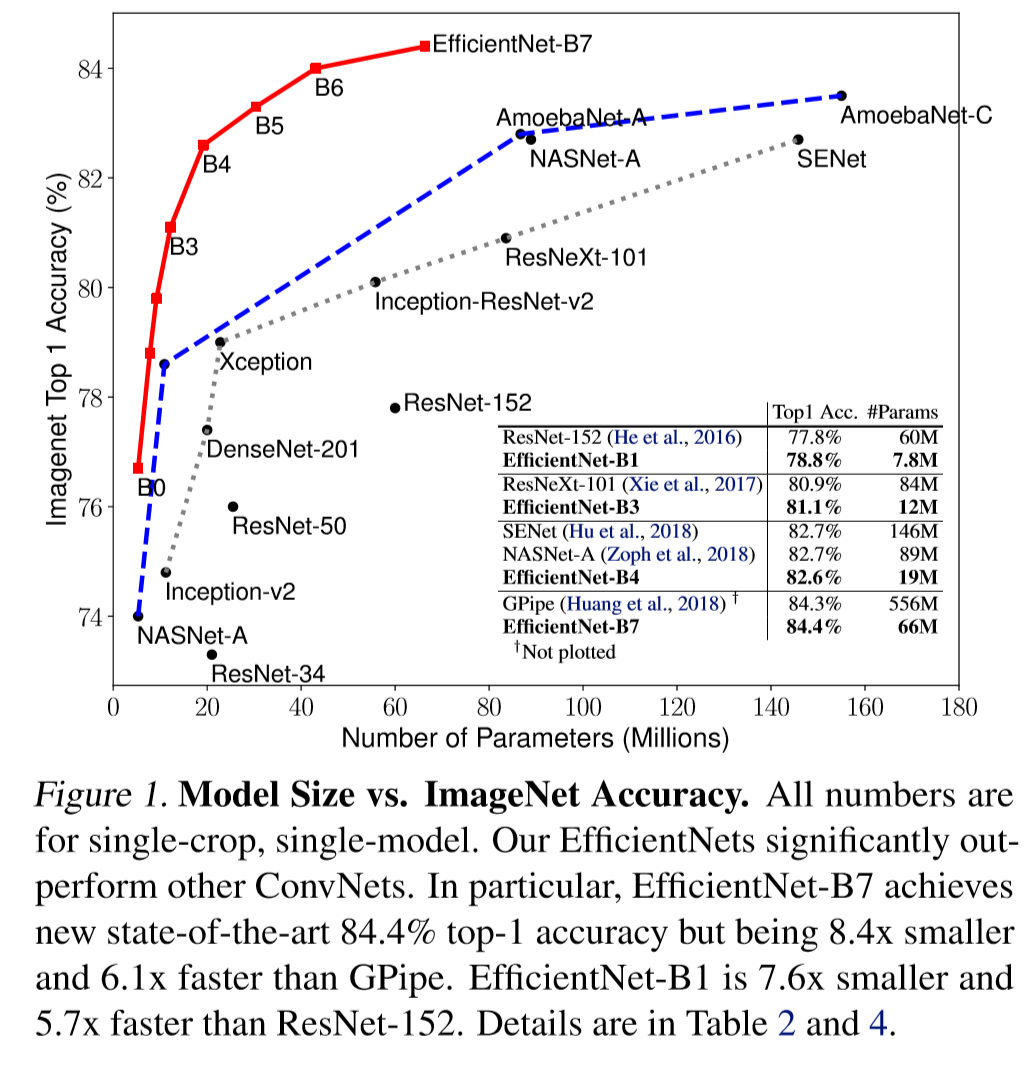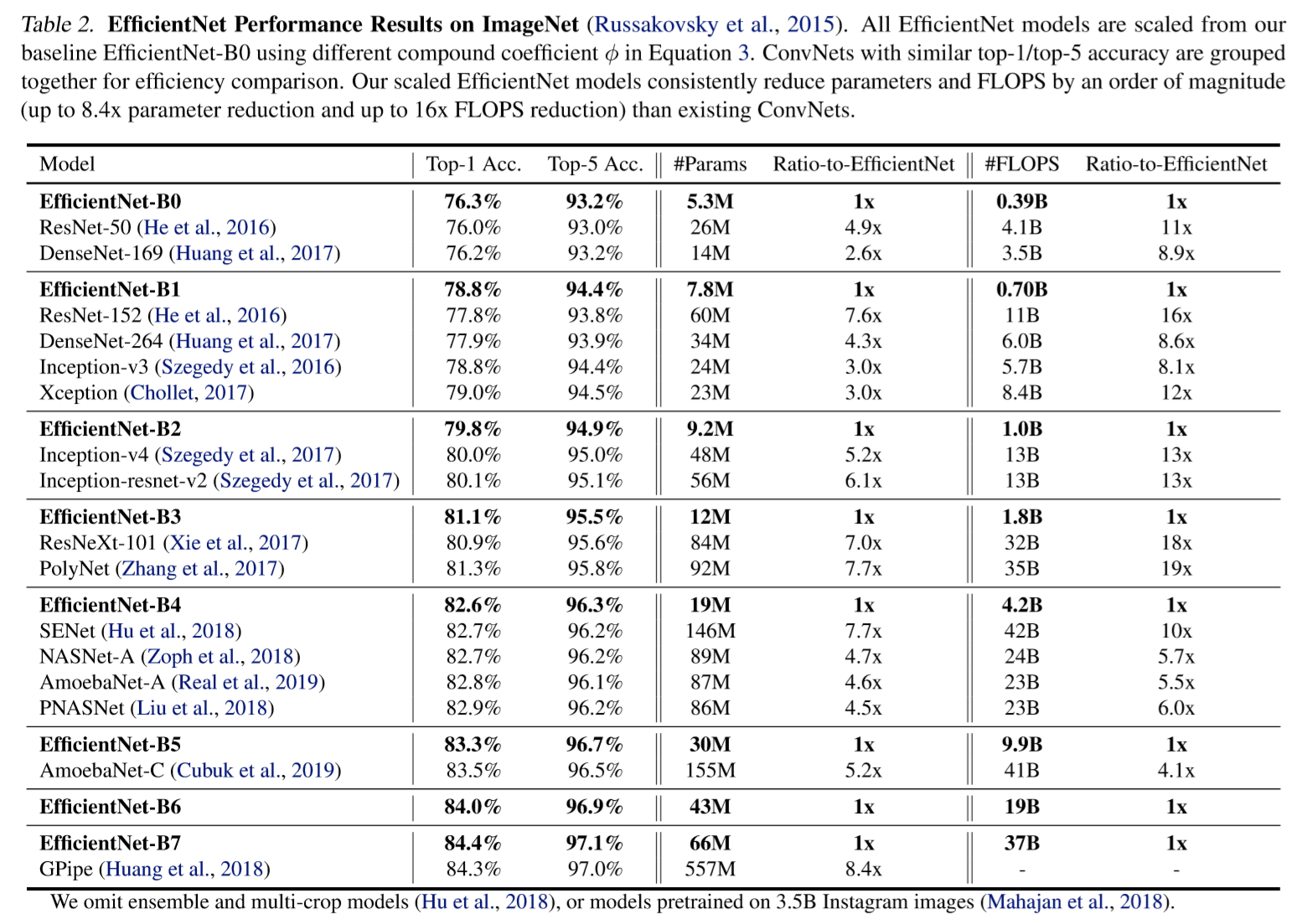Now I will go through two prerequisites, ‘neural architecture search’ and ‘mobilenet-v2’ briefly.

## 2. Neural Architecture Search (NAS)

NAS is an algorithm that finds optimal network architecture. It assumes that the neural net is composed of certain blocks. (ex. 1x7 then 7x1 convolution, 3x3 average pooling, etc) A RNN controller outputs the blocks to build a neural network, then based on it’s validation score on a dataset, the controller is reinforced.

Advanced algorithms such as Progressive NAS and Efficient NAS were invented to significantly reduce search runtime.

Below is an example of cells proposed by NAS.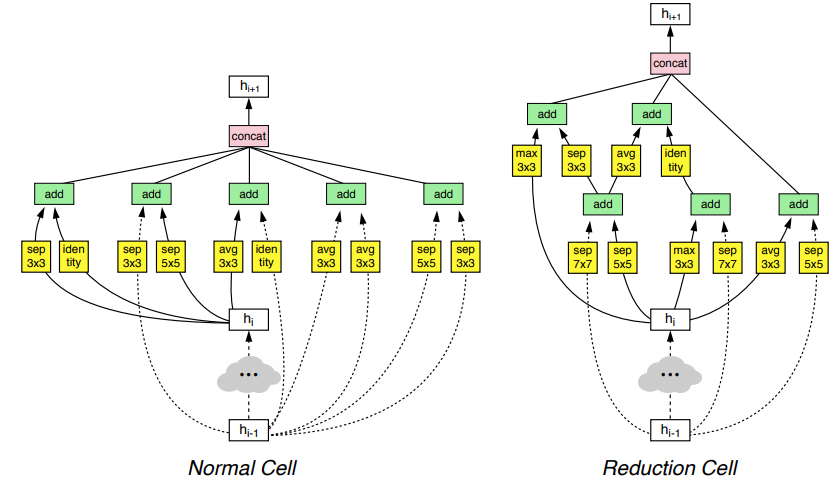## 3. MBConv Block in MobileNet V2

More info about MobileNet v2 here. MobileNet V2 is composed of many MBConv blocks. The blocks have 3 features.

1. Depthwise Convolution + Pointwise Convolution It divides original convolution to two steps to reduce computational cost significantly with minimal accuracy loss.
2. Inverted Residuals Original ResNet blocks are composed with a layer that squeezes channels, then a layer that expands channels, such that skip connection connects rich-channel layers. But in MBConv, blocks are composed with a layer that first expands channels, then squeeze them, so layers that have less channels are skip-connected.
3. Linear Bottleneck It uses linear activation in the last layer in each block to prevent information loss from ReLU.

Below is a keras pseudo code for MBConv block.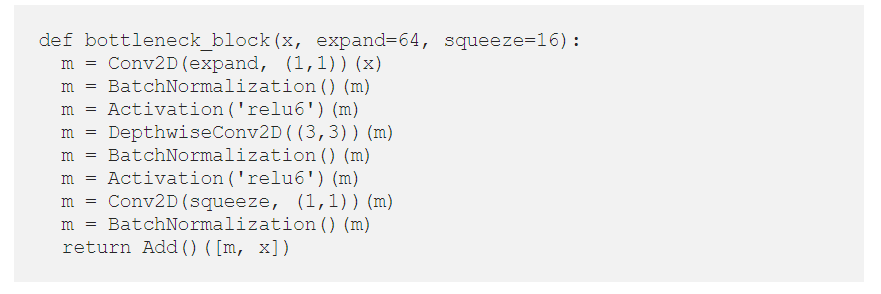## 4. Background

There has been consistent development in ConvNet accuracy since AlexNet(2012), but because of hardware limits, ‘efficiency’ started to gather interest.

Movile-size ConvNets such as SqueezeNets, MobileNets, and ShuffleNets were invented and Neural Architecture Search was widely used. To find out an efficient model structure, one can first search it with a small model, then scale the found architecture to get a bigger model. Typical example is ResNets.

This ‘scaling’ of ConvNets were done on 3 aspects; ‘depth’, ‘width’, and ‘image size’. Conventionally, scaling was done on one of these aspects, but in EfficientNet three components are scaled simultaneously.

Intuitively, when image resolution is increased, the model should need more layers and more filters to extract features from the increased pixels(information). So it seems efficient to scale 3 components at the same time.

Below is an image describing 3 aspects of scaling, and compound scaling which scales 3 components simultaneously.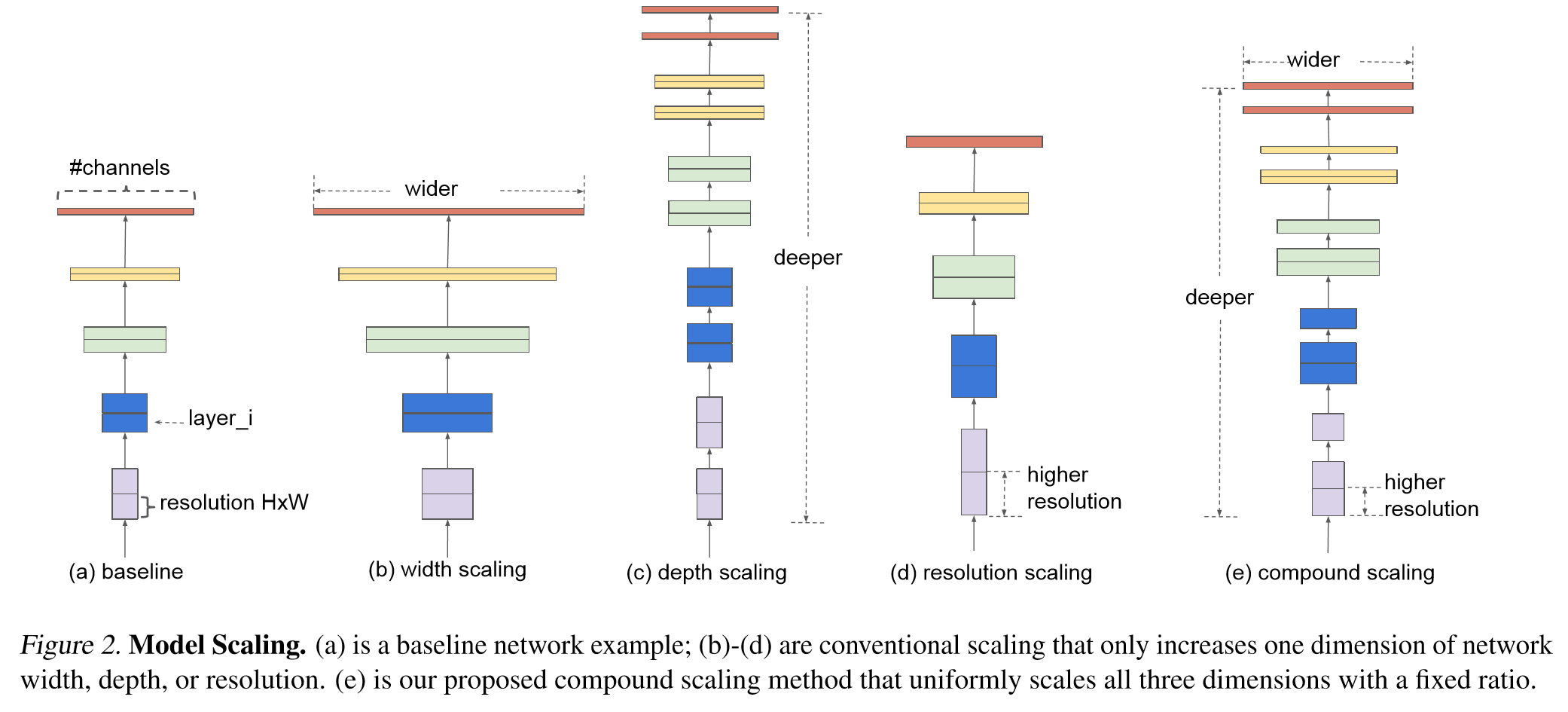## 5. Compound Scaling

The authors observed that it is more effective to scale width, depth, and resolution at the same time, than to scale them separately.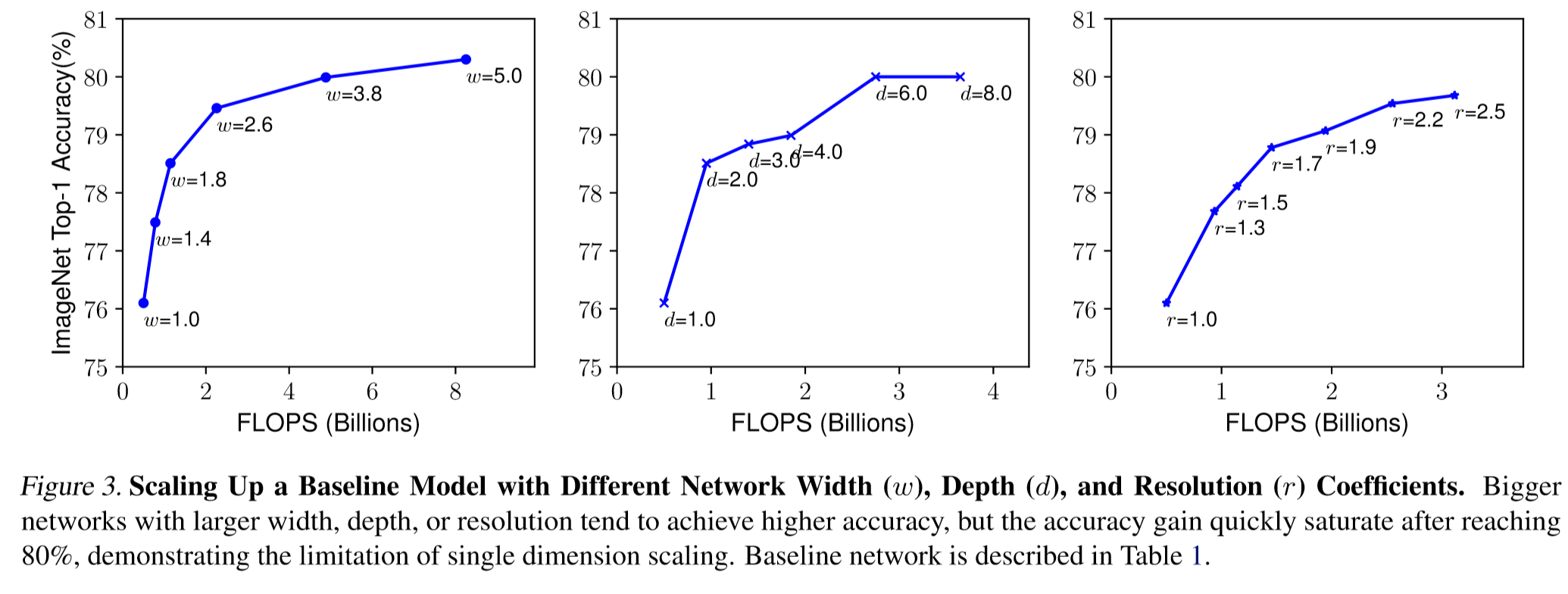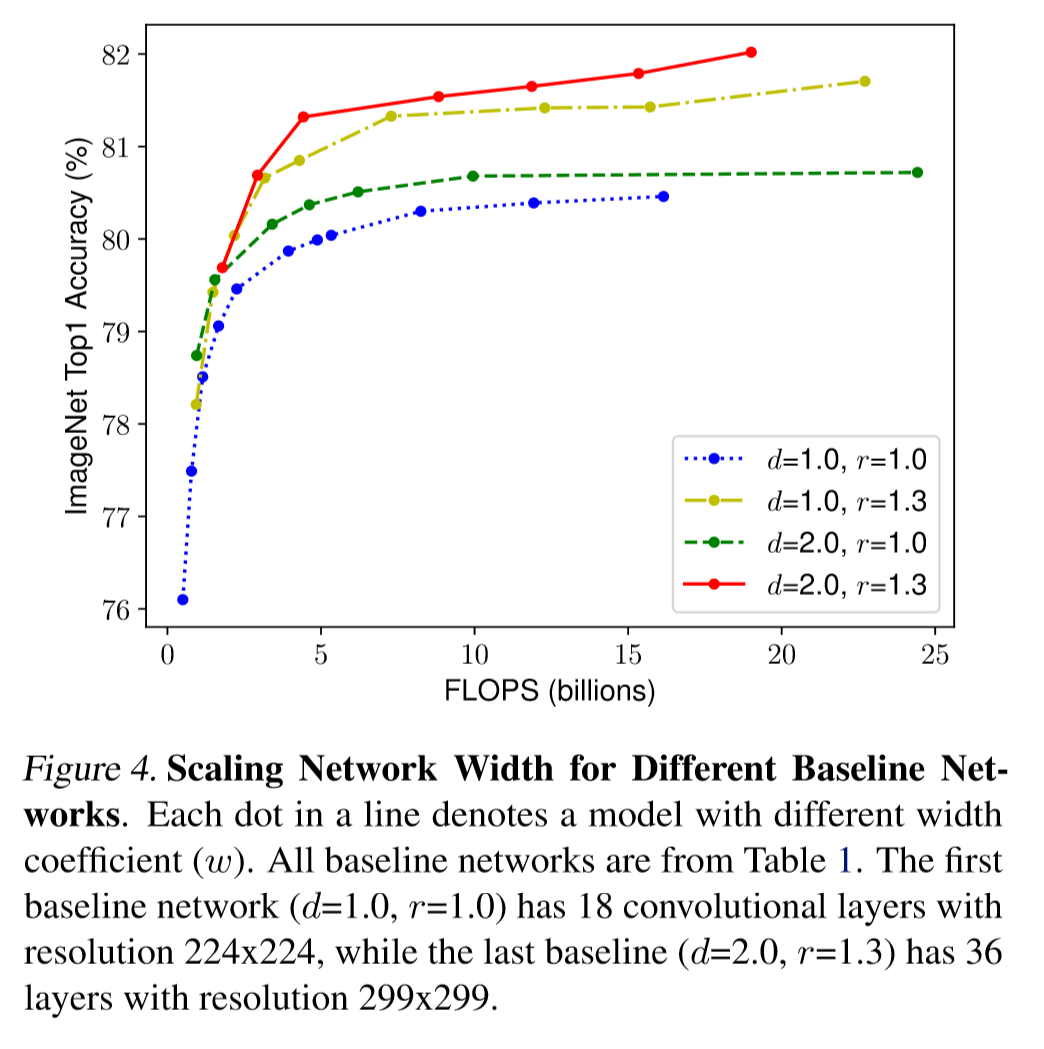The technique is called ‘compound scaling’.

The authors wanted to increase FLOPS by a factor of 2, from EfficientNetB0 to EfficientNetB7. (To make EfficientNetB1 have 2x FLOPS compared to EfficientNetB0, etc) so they formulated the problem like below.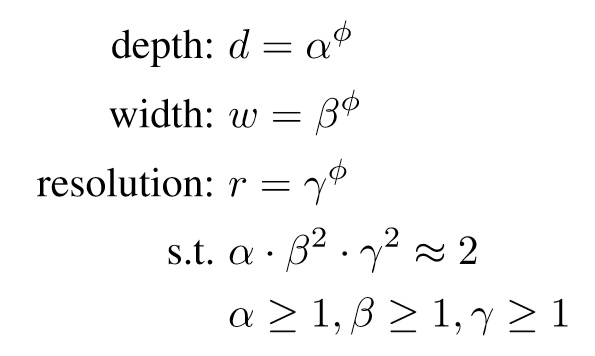where $\phi$ is the suffix,(eg. 2 from EfficientNetB2) and $d,w,r$ is a factor that is multiplied to the base model’s depth, width and resolution respectively.

## 6. Constructing EfficientNet

The authors performed NAS to find an optimal baseline network, EfficientNetB0. It used mobile inverted bottleneck MBConv.

Below is a EfficientNetB0 baseline network.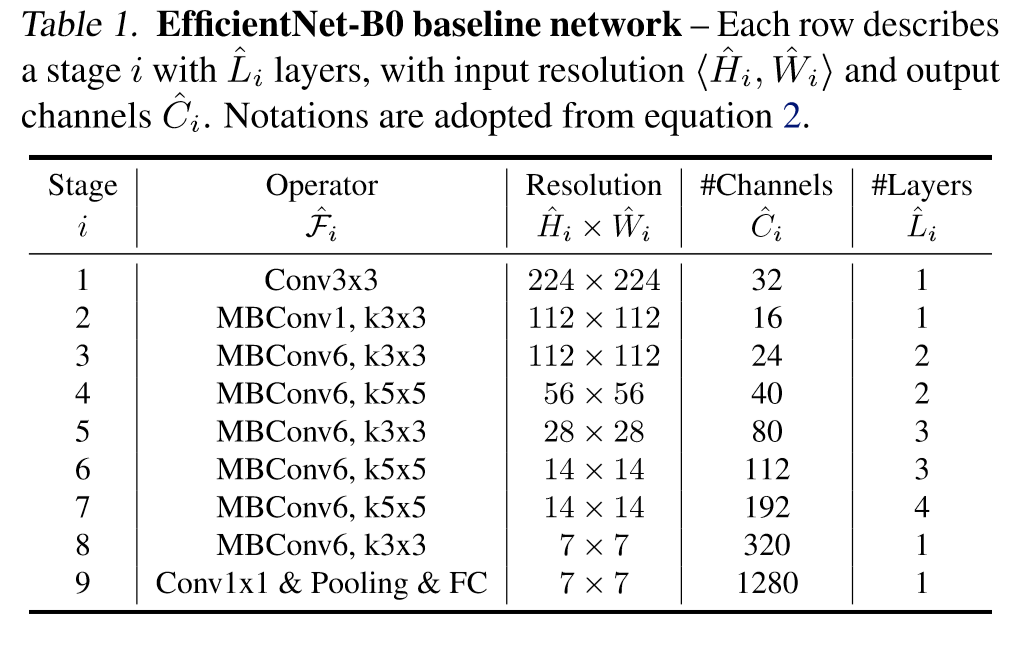### Step 2

Fix $\phi=1$, then grid search $\alpha, \beta, \gamma$ to scale b0 to b1.

### Step 3

Fix $\alpha, \beta, \gamma$, then increase $\phi$ from 2~7 to construct b2~b7 with the compound scaling method.

## 7. Etc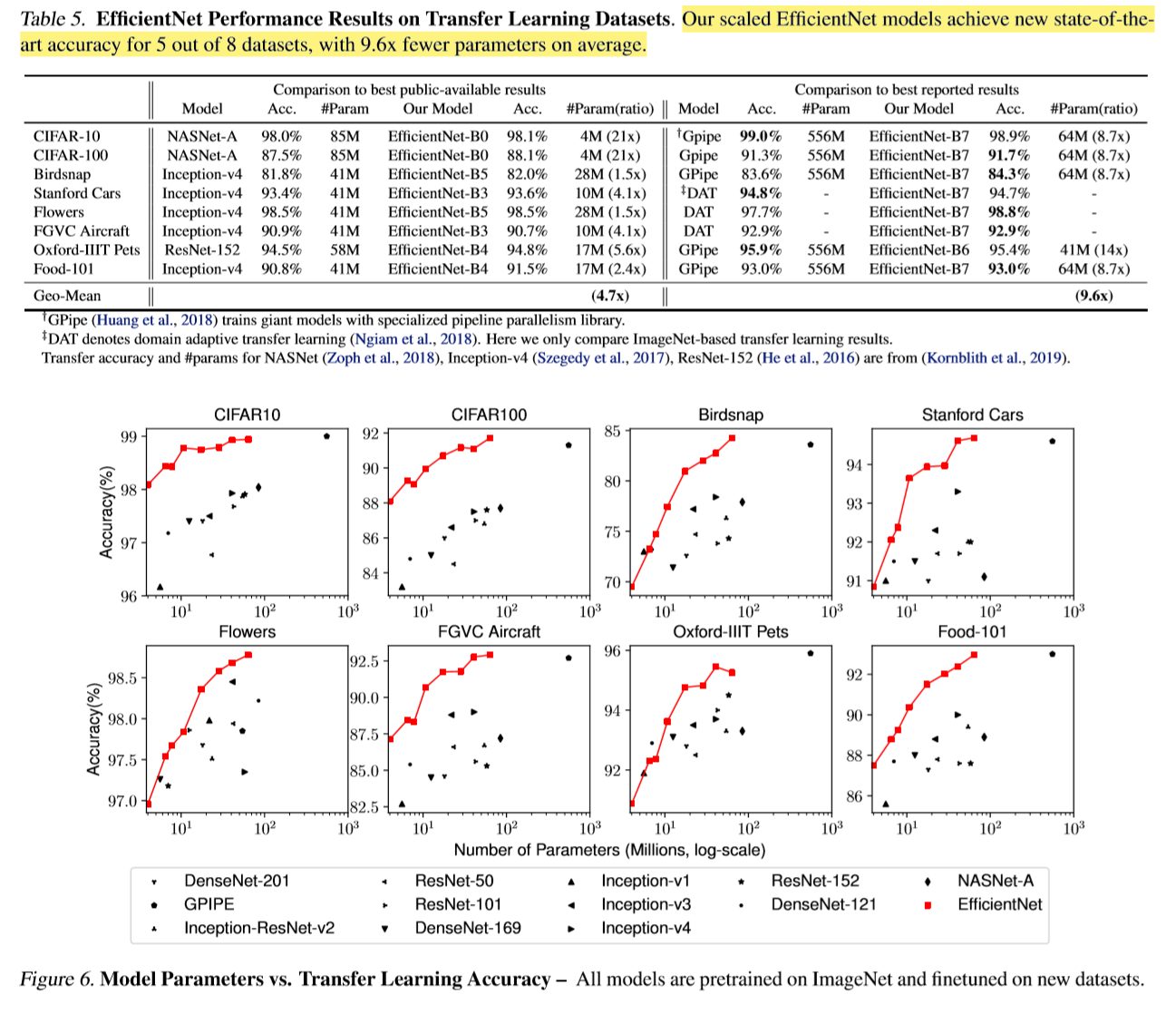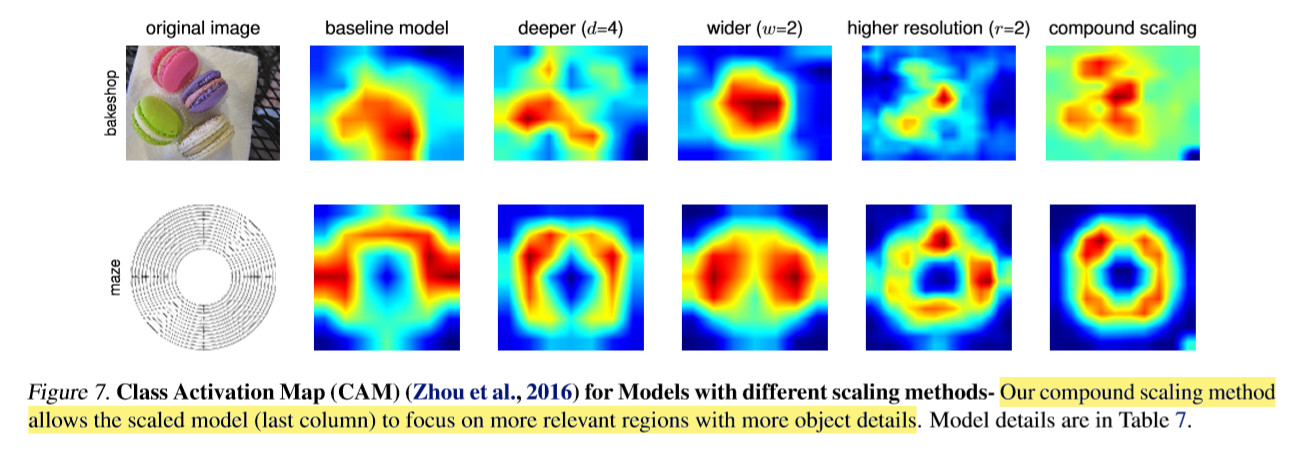Categories: